# 10.4 Polar coordinates: graphs  (Page 7/16)

 Page 7 / 16

Sketch the graph of $\text{\hspace{0.17em}}r=-\theta \text{\hspace{0.17em}}$ over the interval $\text{\hspace{0.17em}}\left[0,4\pi \right].$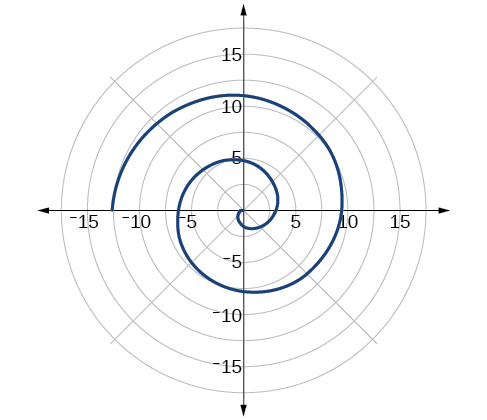## Summary of curves

We have explored a number of seemingly complex polar curves in this section. [link] and [link] summarize the graphs and equations for each of these curves.

Access these online resources for additional instruction and practice with graphs of polar coordinates.

## Key concepts

• It is easier to graph polar equations if we can test the equations for symmetry with respect to the line $\text{\hspace{0.17em}}\theta =\frac{\pi }{2},\text{\hspace{0.17em}}$ the polar axis, or the pole.
• There are three symmetry tests that indicate whether the graph of a polar equation will exhibit symmetry. If an equation fails a symmetry test, the graph may or may not exhibit symmetry. See [link] .
• Polar equations may be graphed by making a table of values for $\text{\hspace{0.17em}}\theta \text{\hspace{0.17em}}$ and $\text{\hspace{0.17em}}r.$
• The maximum value of a polar equation is found by substituting the value $\text{\hspace{0.17em}}\theta \text{\hspace{0.17em}}$ that leads to the maximum value of the trigonometric expression.
• The zeros of a polar equation are found by setting $\text{\hspace{0.17em}}r=0\text{\hspace{0.17em}}$ and solving for $\text{\hspace{0.17em}}\theta .\text{\hspace{0.17em}}$ See [link] .
• Some formulas that produce the graph of a circle in polar coordinates are given by $\text{\hspace{0.17em}}r=a\mathrm{cos}\text{\hspace{0.17em}}\theta \text{\hspace{0.17em}}$ and $\text{\hspace{0.17em}}r=a\mathrm{sin}\text{\hspace{0.17em}}\theta .\text{\hspace{0.17em}}$ See [link] .
• The formulas that produce the graphs of a cardioid are given by $\text{\hspace{0.17em}}r=a±b\mathrm{cos}\text{\hspace{0.17em}}\theta \text{\hspace{0.17em}}$ and $\text{\hspace{0.17em}}r=a±b\mathrm{sin}\text{\hspace{0.17em}}\theta ,\text{\hspace{0.17em}}$ for $\text{\hspace{0.17em}}a>0,\text{\hspace{0.17em}}\text{\hspace{0.17em}}b>0,\text{\hspace{0.17em}}$ and $\text{\hspace{0.17em}}\frac{a}{b}=1.\text{\hspace{0.17em}}$ See [link] .
• The formulas that produce the graphs of a one-loop limaçon are given by $\text{\hspace{0.17em}}r=a±b\mathrm{cos}\text{\hspace{0.17em}}\theta \text{\hspace{0.17em}}$ and $\text{\hspace{0.17em}}r=a±b\mathrm{sin}\text{\hspace{0.17em}}\theta \text{\hspace{0.17em}}$ for $\text{\hspace{0.17em}}1<\frac{a}{b}<2.\text{\hspace{0.17em}}$ See [link] .
• The formulas that produce the graphs of an inner-loop limaçon are given by $\text{\hspace{0.17em}}r=a±b\mathrm{cos}\text{\hspace{0.17em}}\theta \text{\hspace{0.17em}}$ and $\text{\hspace{0.17em}}r=a±b\mathrm{sin}\text{\hspace{0.17em}}\theta \text{\hspace{0.17em}}$ for $\text{\hspace{0.17em}}a>0,\text{\hspace{0.17em}}\text{\hspace{0.17em}}b>0,\text{\hspace{0.17em}}$ and $\text{\hspace{0.17em}}a See [link] .
• The formulas that produce the graphs of a lemniscates are given by $\text{\hspace{0.17em}}{r}^{2}={a}^{2}\mathrm{cos}\text{\hspace{0.17em}}2\theta \text{\hspace{0.17em}}$ and $\text{\hspace{0.17em}}{r}^{2}={a}^{2}\mathrm{sin}\text{\hspace{0.17em}}2\theta ,\text{\hspace{0.17em}}$ where $\text{\hspace{0.17em}}a\ne 0.$ See [link] .
• The formulas that produce the graphs of rose curves are given by $\text{\hspace{0.17em}}r=a\mathrm{cos}\text{\hspace{0.17em}}n\theta \text{\hspace{0.17em}}$ and $\text{\hspace{0.17em}}r=a\mathrm{sin}\text{\hspace{0.17em}}n\theta ,\text{\hspace{0.17em}}$ where $\text{\hspace{0.17em}}a\ne 0;\text{\hspace{0.17em}}$ if $\text{\hspace{0.17em}}n\text{\hspace{0.17em}}$ is even, there are $\text{\hspace{0.17em}}2n\text{\hspace{0.17em}}$ petals, and if $\text{\hspace{0.17em}}n\text{\hspace{0.17em}}$ is odd, there are $\text{\hspace{0.17em}}n\text{\hspace{0.17em}}$ petals. See [link] and [link] .
• The formula that produces the graph of an Archimedes’ spiral is given by $\text{\hspace{0.17em}}r=\theta ,\text{\hspace{0.17em}}\text{\hspace{0.17em}}\theta \ge 0.\text{\hspace{0.17em}}$ See [link] .

## Verbal

Describe the three types of symmetry in polar graphs, and compare them to the symmetry of the Cartesian plane.

Symmetry with respect to the polar axis is similar to symmetry about the $\text{\hspace{0.17em}}x$ -axis, symmetry with respect to the pole is similar to symmetry about the origin, and symmetric with respect to the line $\text{\hspace{0.17em}}\theta =\frac{\pi }{2}\text{\hspace{0.17em}}$ is similar to symmetry about the $\text{\hspace{0.17em}}y$ -axis.

Which of the three types of symmetries for polar graphs correspond to the symmetries with respect to the x -axis, y -axis, and origin?

What are the steps to follow when graphing polar equations?

Test for symmetry; find zeros, intercepts, and maxima; make a table of values. Decide the general type of graph, cardioid, limaçon, lemniscate, etc., then plot points at and sketch the graph.

Describe the shapes of the graphs of cardioids, limaçons, and lemniscates.

What part of the equation determines the shape of the graph of a polar equation?

The shape of the polar graph is determined by whether or not it includes a sine, a cosine, and constants in the equation.

## Graphical

For the following exercises, test the equation for symmetry.

$r=5\mathrm{cos}\text{\hspace{0.17em}}3\theta$

$r=3-3\mathrm{cos}\text{\hspace{0.17em}}\theta$

symmetric with respect to the polar axis

$r=3+2\mathrm{sin}\text{\hspace{0.17em}}\theta$

$r=3\mathrm{sin}\text{\hspace{0.17em}}2\theta$

symmetric with respect to the polar axis, symmetric with respect to the line $\theta =\frac{\pi }{2},$ symmetric with respect to the pole

$r=4$

$r=2\theta$

no symmetry

$r=4\mathrm{cos}\text{\hspace{0.17em}}\frac{\theta }{2}$

$r=\frac{2}{\theta }$

no symmetry

$r=3\sqrt{1-{\mathrm{cos}}^{2}\theta }$

$r=\sqrt{5\mathrm{sin}\text{\hspace{0.17em}}2\theta }$

symmetric with respect to the pole

For the following exercises, graph the polar equation. Identify the name of the shape.

$r=3\mathrm{cos}\text{\hspace{0.17em}}\theta$

$r=4\mathrm{sin}\text{\hspace{0.17em}}\theta$

circle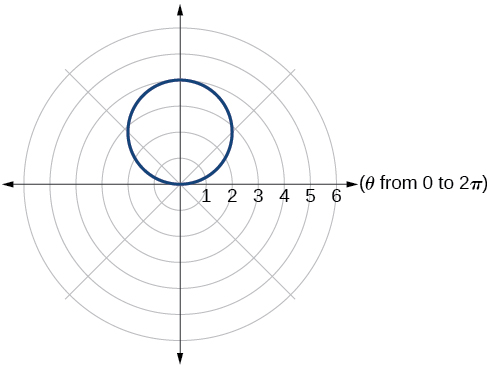$r=2+2\mathrm{cos}\text{\hspace{0.17em}}\theta$

$r=2-2\mathrm{cos}\text{\hspace{0.17em}}\theta$

cardioid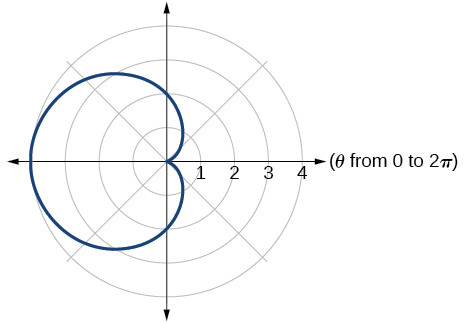$r=5-5\mathrm{sin}\text{\hspace{0.17em}}\theta$

$r=3+3\mathrm{sin}\text{\hspace{0.17em}}\theta$

cardioid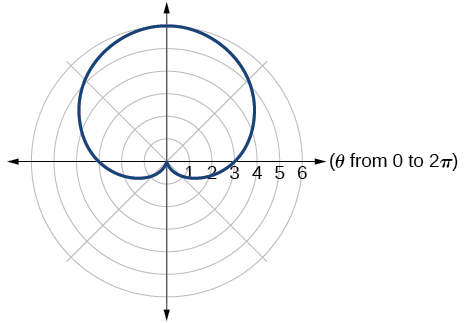$r=3+2\mathrm{sin}\text{\hspace{0.17em}}\theta$

$r=7+4\mathrm{sin}\text{\hspace{0.17em}}\theta$

one-loop/dimpled limaçon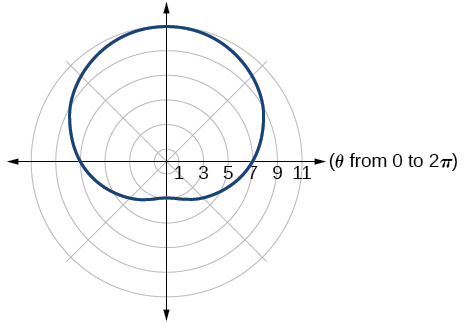$r=4+3\mathrm{cos}\text{\hspace{0.17em}}\theta$

$r=5+4\mathrm{cos}\text{\hspace{0.17em}}\theta$

one-loop/dimpled limaçon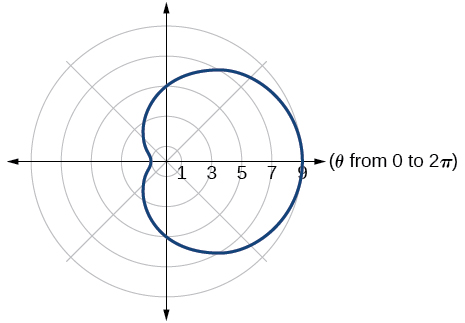$r=10+9\mathrm{cos}\text{\hspace{0.17em}}\theta$

$r=1+3\mathrm{sin}\text{\hspace{0.17em}}\theta$

inner loop/two-loop limaçon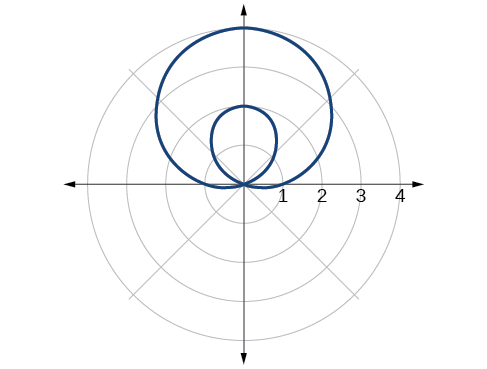$r=2+5\mathrm{sin}\text{\hspace{0.17em}}\theta$

$r=5+7\mathrm{sin}\text{\hspace{0.17em}}\theta$

inner loop/two-loop limaçon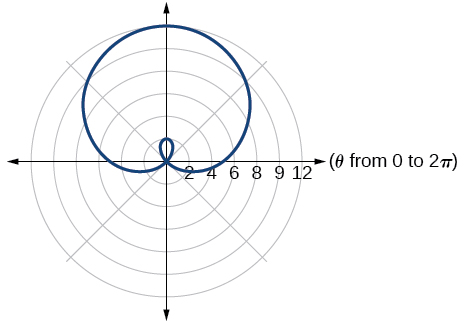$r=2+4\mathrm{cos}\text{\hspace{0.17em}}\theta$

$r=5+6\mathrm{cos}\text{\hspace{0.17em}}\theta$

inner loop/two-loop limaçon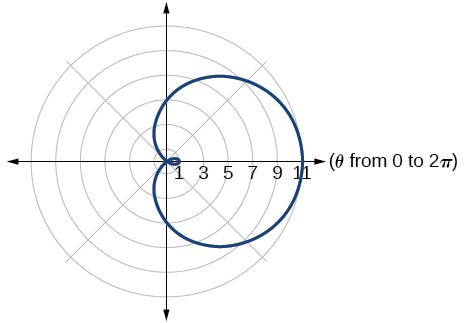${r}^{2}=36\mathrm{cos}\left(2\theta \right)$

${r}^{2}=10\mathrm{cos}\left(2\theta \right)$

lemniscate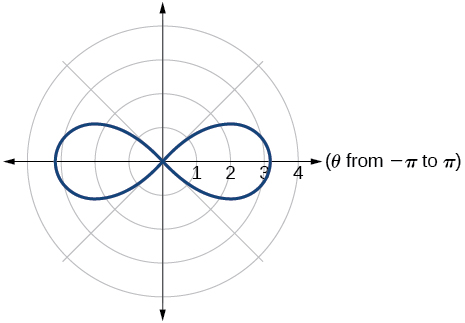${r}^{2}=4\mathrm{sin}\left(2\theta \right)$

${r}^{2}=10\mathrm{sin}\left(2\theta \right)$

lemniscate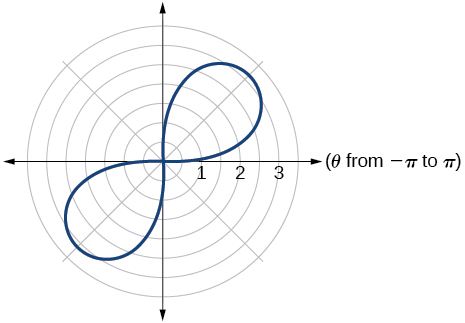$r=3\text{sin}\left(2\theta \right)$

$r=3\text{cos}\left(2\theta \right)$

rose curve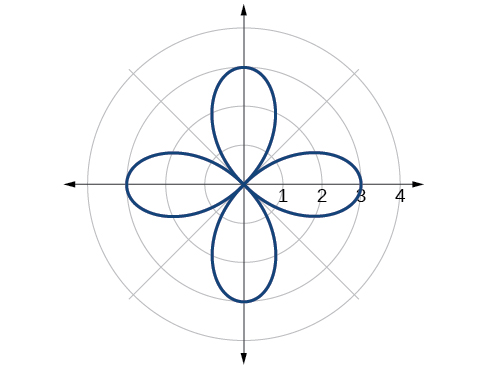$r=5\text{sin}\left(3\theta \right)$

$r=4\text{sin}\left(4\theta \right)$

rose curve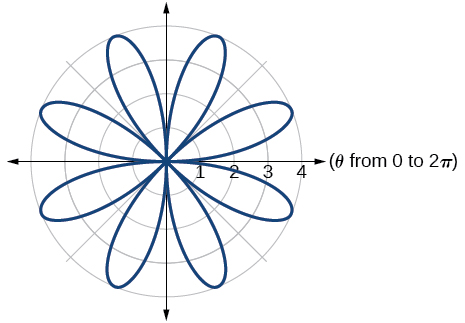$r=4\text{sin}\left(5\theta \right)$

$r=-\theta$

Archimedes’ spiral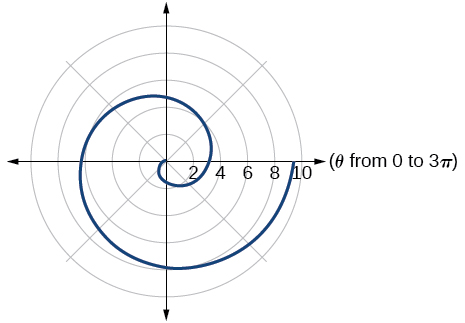$r=2\theta$

$r=-3\theta$

Archimedes’ spiral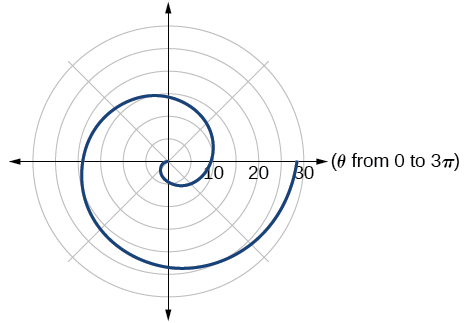## Technology

For the following exercises, use a graphing calculator to sketch the graph of the polar equation.

$r=\frac{1}{\theta }$

$r=\frac{1}{\sqrt{\theta }}$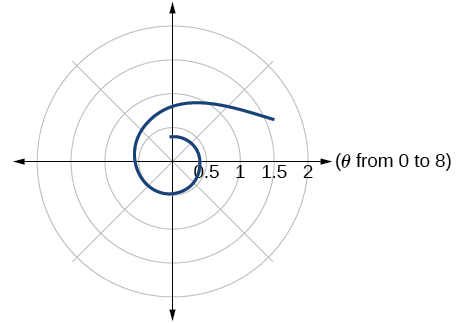$r=2\mathrm{sin}\text{\hspace{0.17em}}\theta \mathrm{tan}\text{\hspace{0.17em}}\theta ,$ a cissoid

$r=2\sqrt{1-{\mathrm{sin}}^{2}\theta }$ , a hippopede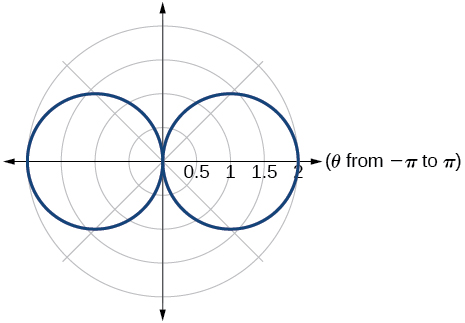$r=5+\mathrm{cos}\left(4\theta \right)$

$r=2-\mathrm{sin}\left(2\theta \right)$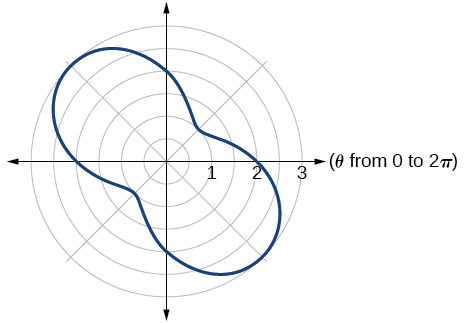$r={\theta }^{2}$

$r=\theta +1$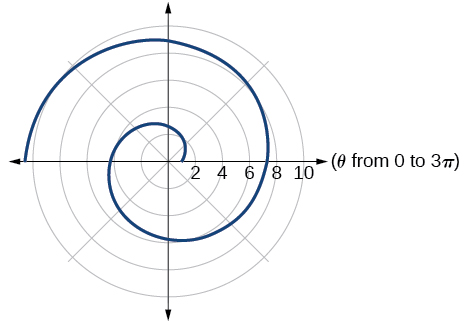$r=\theta \mathrm{sin}\text{\hspace{0.17em}}\theta$

$r=\theta \mathrm{cos}\text{\hspace{0.17em}}\theta$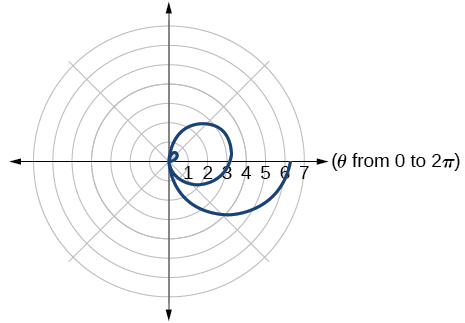For the following exercises, use a graphing utility to graph each pair of polar equations on a domain of $\text{\hspace{0.17em}}\left[0,4\pi \right]\text{\hspace{0.17em}}$ and then explain the differences shown in the graphs.

$r=\theta ,r=-\theta$

$r=\theta ,r=\theta +\mathrm{sin}\text{\hspace{0.17em}}\theta$

They are both spirals, but not quite the same.

$r=\mathrm{sin}\text{\hspace{0.17em}}\theta +\theta ,r=\mathrm{sin}\text{\hspace{0.17em}}\theta -\theta$

$r=2\mathrm{sin}\left(\frac{\theta }{2}\right),r=\theta \mathrm{sin}\left(\frac{\theta }{2}\right)$

Both graphs are curves with 2 loops. The equation with a coefficient of $\text{\hspace{0.17em}}\theta \text{\hspace{0.17em}}$ has two loops on the left, the equation with a coefficient of 2 has two loops side by side. Graph these from 0 to $\text{\hspace{0.17em}}4\pi \text{\hspace{0.17em}}$ to get a better picture.

$r=\mathrm{sin}\left(\mathrm{cos}\left(3\theta \right)\right)\text{\hspace{0.17em}}\text{\hspace{0.17em}}r=\mathrm{sin}\left(3\theta \right)$

On a graphing utility, graph $\text{\hspace{0.17em}}r=\mathrm{sin}\left(\frac{16}{5}\theta \right)\text{\hspace{0.17em}}$ on $\text{\hspace{0.17em}}\left[0,4\pi \right],\left[0,8\pi \right],\left[0,12\pi \right],\text{\hspace{0.17em}}$ and $\text{\hspace{0.17em}}\left[0,16\pi \right].\text{\hspace{0.17em}}$ Describe the effect of increasing the width of the domain.

When the width of the domain is increased, more petals of the flower are visible.

On a graphing utility, graph and sketch $\text{\hspace{0.17em}}r=\mathrm{sin}\text{\hspace{0.17em}}\theta +{\left(\mathrm{sin}\left(\frac{5}{2}\theta \right)\right)}^{3}\text{\hspace{0.17em}}$ on $\text{\hspace{0.17em}}\left[0,4\pi \right].$

On a graphing utility, graph each polar equation. Explain the similarities and differences you observe in the graphs.

$\begin{array}{l}\begin{array}{l}\\ {r}_{1}=3\mathrm{sin}\left(3\theta \right)\end{array}\hfill \\ {r}_{2}=2\mathrm{sin}\left(3\theta \right)\hfill \\ {r}_{3}=\mathrm{sin}\left(3\theta \right)\hfill \end{array}$

The graphs are three-petal, rose curves. The larger the coefficient, the greater the curve’s distance from the pole.

On a graphing utility, graph each polar equation. Explain the similarities and differences you observe in the graphs.

$\begin{array}{l}\begin{array}{l}\\ {r}_{1}=3+3\mathrm{cos}\text{\hspace{0.17em}}\theta \end{array}\hfill \\ {r}_{2}=2+2\mathrm{cos}\text{\hspace{0.17em}}\theta \hfill \\ {r}_{3}=1+\mathrm{cos}\text{\hspace{0.17em}}\theta \hfill \end{array}$

On a graphing utility, graph each polar equation. Explain the similarities and differences you observe in the graphs.

$\begin{array}{l}\begin{array}{l}\\ {r}_{1}=3\theta \end{array}\hfill \\ {r}_{2}=2\theta \hfill \\ {r}_{3}=\theta \hfill \end{array}$

The graphs are spirals. The smaller the coefficient, the tighter the spiral.

## Extensions

For the following exercises, draw each polar equation on the same set of polar axes, and find the points of intersection.

${r}_{1}=3+2\mathrm{sin}\text{\hspace{0.17em}}\theta ,\text{\hspace{0.17em}}{r}_{2}=2$

${r}_{1}=6-4\mathrm{cos}\text{\hspace{0.17em}}\theta ,\text{\hspace{0.17em}}{r}_{2}=4$

$\left(4,\frac{\pi }{3}\right),\left(4,\frac{5\pi }{3}\right)$

${r}_{1}=1+\mathrm{sin}\text{\hspace{0.17em}}\theta ,\text{\hspace{0.17em}}{r}_{2}=3\mathrm{sin}\text{\hspace{0.17em}}\theta$

${r}_{1}=1+\mathrm{cos}\text{\hspace{0.17em}}\theta ,\text{\hspace{0.17em}}{r}_{2}=3\mathrm{cos}\text{\hspace{0.17em}}\theta$

$\left(\frac{3}{2},\frac{\pi }{3}\right),\left(\frac{3}{2},\frac{5\pi }{3}\right)$

${r}_{1}=\mathrm{cos}\left(2\theta \right),\text{\hspace{0.17em}}{r}_{2}=\mathrm{sin}\left(2\theta \right)$

${r}_{1}={\mathrm{sin}}^{2}\left(2\theta \right),\text{\hspace{0.17em}}{r}_{2}=1-\mathrm{cos}\left(4\theta \right)$

$\left(0,\frac{\pi }{2}\right),\text{\hspace{0.17em}}\left(0,\pi \right),\text{\hspace{0.17em}}\left(0,\frac{3\pi }{2}\right),\text{\hspace{0.17em}}\left(0,2\pi \right)$

${r}_{1}=\sqrt{3},\text{\hspace{0.17em}}{r}_{2}=2\mathrm{sin}\left(\theta \right)$

${r}_{1}{}^{2}=\mathrm{sin}\text{\hspace{0.17em}}\theta ,{r}_{2}{}^{2}=\mathrm{cos}\text{\hspace{0.17em}}\theta$

$\left(\frac{\sqrt{8}}{2},\frac{\pi }{4}\right),\text{\hspace{0.17em}}\left(\frac{\sqrt{8}}{2},\frac{5\pi }{4}\right)\text{\hspace{0.17em}}$ and at $\text{\hspace{0.17em}}\theta =\frac{3\pi }{4},\text{\hspace{0.17em}}\text{\hspace{0.17em}}\frac{7\pi }{4}\text{\hspace{0.17em}}\text{\hspace{0.17em}}$ since $\text{\hspace{0.17em}}r\text{\hspace{0.17em}}$ is squared

${r}_{1}=1+\mathrm{cos}\text{\hspace{0.17em}}\theta ,\text{\hspace{0.17em}}{r}_{2}=1-\mathrm{sin}\text{\hspace{0.17em}}\theta$

#### Questions & Answers

A laser rangefinder is locked on a comet approaching Earth. The distance g(x), in kilometers, of the comet after x days, for x in the interval 0 to 30 days, is given by g(x)=250,000csc(π30x). Graph g(x) on the interval [0, 35]. Evaluate g(5)  and interpret the information. What is the minimum distance between the comet and Earth? When does this occur? To which constant in the equation does this correspond? Find and discuss the meaning of any vertical asymptotes.
Kaitlyn Reply
The sequence is {1,-1,1-1.....} has
amit Reply
circular region of radious
Kainat Reply
how can we solve this problem
Joel Reply
Sin(A+B) = sinBcosA+cosBsinA
Eseka Reply
Prove it
Eseka
Please prove it
Eseka
hi
Joel
June needs 45 gallons of punch. 2 different coolers. Bigger cooler is 5 times as large as smaller cooler. How many gallons in each cooler?
Arleathia Reply
7.5 and 37.5
Nando
find the sum of 28th term of the AP 3+10+17+---------
Prince Reply
I think you should say "28 terms" instead of "28th term"
Vedant
the 28th term is 175
Nando
192
Kenneth
if sequence sn is a such that sn>0 for all n and lim sn=0than prove that lim (s1 s2............ sn) ke hole power n =n
SANDESH Reply
write down the polynomial function with root 1/3,2,-3 with solution
Gift Reply
if A and B are subspaces of V prove that (A+B)/B=A/(A-B)
Pream Reply
write down the value of each of the following in surd form a)cos(-65°) b)sin(-180°)c)tan(225°)d)tan(135°)
Oroke Reply
Prove that (sinA/1-cosA - 1-cosA/sinA) (cosA/1-sinA - 1-sinA/cosA) = 4
kiruba Reply
what is the answer to dividing negative index
Morosi Reply
In a triangle ABC prove that. (b+c)cosA+(c+a)cosB+(a+b)cisC=a+b+c.
Shivam Reply
give me the waec 2019 questions
Aaron Reply

### Read also:

#### Get the best Algebra and trigonometry course in your pocket!

Source:  OpenStax, Algebra and trigonometry. OpenStax CNX. Nov 14, 2016 Download for free at https://legacy.cnx.org/content/col11758/1.6
Google Play and the Google Play logo are trademarks of Google Inc.

Notification Switch

Would you like to follow the 'Algebra and trigonometry' conversation and receive update notifications?ByByByBy RhodesBy